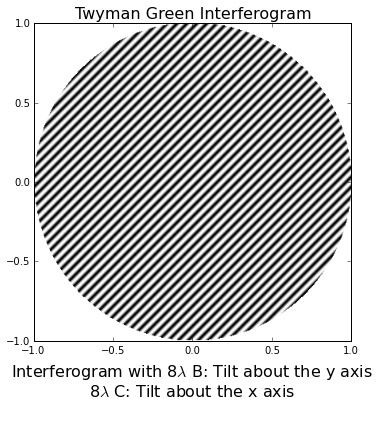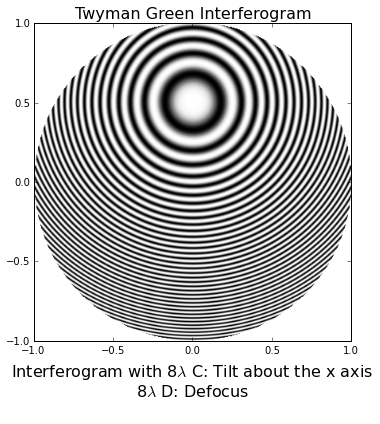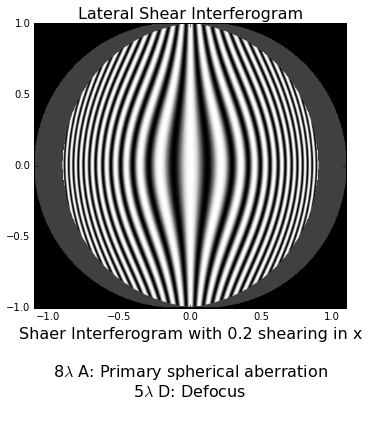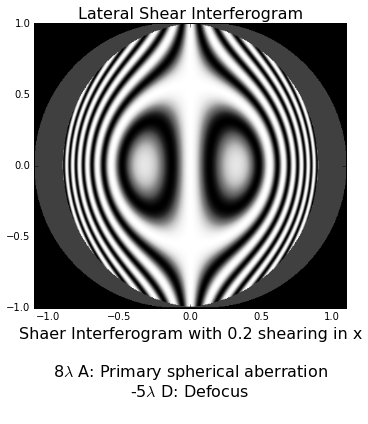## Opticspy can generate serveral kinds interferogram¶

### 4. Other helpful methods to research interferometer and specific help manual¶

In :
%matplotlib inline
import opticspy


### Generate Twyman Green interferogram based on Seidel aberration¶

A: Constant(piston)term
B: Tilt about the y axis
C: Tilt about the x axis
D: Reference sphere change, also called defocus
E: Sagittal astigmatism along the y axis
F: Sagittal coma along the y axis
G: Primary spherical aberration
In :
opticspy.interferometer_seidel.twyman_green(0,8,8,0,0,0,0)In :
opticspy.interferometer_seidel.twyman_green(0,0,8,8,0,0,0)In :
help(opticspy.interferometer_seidel.twyman_green)

Help on function twyman_green in module opticspy.interferometer_seidel:

twyman_green(A=0, B=0, C=0, D=0, E=0, F=0, G=0, lambda_1=632, PR=1)
Genertate Twyman_Green Interferogram based on Seidel aberration
=============================================

input
----------------------------------------------

coefficients in wavenumber(ex. D=8 means 8 max error
in defocus aberration)

A: Constant(piston)term
B: Tilt about the y axis
C: Tilt about the x axis
D: Reference sphere change, also called defocus
E: Sagittal astigmatism along the y axis
F: Sagittal coma along the y axis
G: Primary spherical aberration
lambda_1: wavelength in nanometer, default = 632nm
PR: pupil radius, default = 1

output
----------------------------------------------
Interferogram of aberration



### Generate lateral shear interferogram based on Seidel aberration.¶

In :
opticspy.interferometer_seidel.lateral_shear(8,0,0,5,0,0.2)In :
opticspy.interferometer_seidel.lateral_shear(8,0,0,-5,0,0.2)In :
help(opticspy.interferometer_seidel.lateral_shear)

Help on function lateral_shear in module opticspy.interferometer_seidel:

lateral_shear(A=0, B=0, C=0, D=0, E=0, S=0.1, lambda_1=632, PR=1)
Genertate Lateral_Shear Interferogram
=============================================

input
Lateral_Shear(A, B, C, D, E, S, lambda_1 = 632, PR = 1):
----------------------------------------------

coefficients in wavenumber(ex. D=8 means 8 max error
in defocus aberration)

A: Primary spherical aberration
B: Coma
C: Astigmatism
D: Defocus
E: x-Tilt
S: Shear distance(positive)
lambda_1: wavelength in nanometer, default = 632nm
PR: pupil radius, default = 1

output
----------------------------------------------
Lateral Shear interferogram of aberration



### Generate Twyman Green Interferogram based on Zernike polynomials¶

In :
Z = opticspy.zernike.Coefficient(Z2=1,Z4=0.5,Z11=1)

In :
Z.listcoefficient()

Z2 =  1 Z11 x Tilt
Z4 =  0.5 Z20 Defocus
Z11 =  1 Z40 Primary Spherical

Out:
['Z2 = 1\nZ4 = 0.5\nZ11 = 1\n', 'Z2 = 1  Z4 = 0.5  Z11 = 1  ']
In :
opticspy.interferometer_zenike.twyman_green(Z)